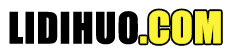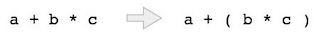# 表达式解析

Infix 符号 Prefix（Polish）符号 Postfix（Reverse-Polish）符号

## Postfix符号

 Infix Prefix Postfix a + b + a b a b + (a + b) * c * + a b c a b + c * a * (b + c) * a + b c a b c + * a/b + c/d +/a b/c d a b/c d/+ (a + b) * (c + d) * + a b + c d a b + c d + * ((a + b) * c)-d -* + a b c d a b + c * d-

## 解析表达式

### 优先级### 关联性

 运算符 优先级 关联性 求幂^ 最高 右结合 乘法（*）和除法（/） 第二高 左联想 加法（+）和减法（-） 最低 左联想

a + b*c 中，表达式部分 b* c 将首先被计算，乘法优先于加法。我们在这里使用括号来首先评估 a + b，例如 (a + b)*c

## 后缀评估算法

```Step 1 − scan the expression from left to right
Step 2 − if it is an operand push it to stack
Step 3 − if it is an operator pull operand from stack and perform operation
Step 4 − store the output of step 3, back to stack
Step 5 − scan the expression until all operands are consumed
Step 6 − pop the stack and perform operation
```